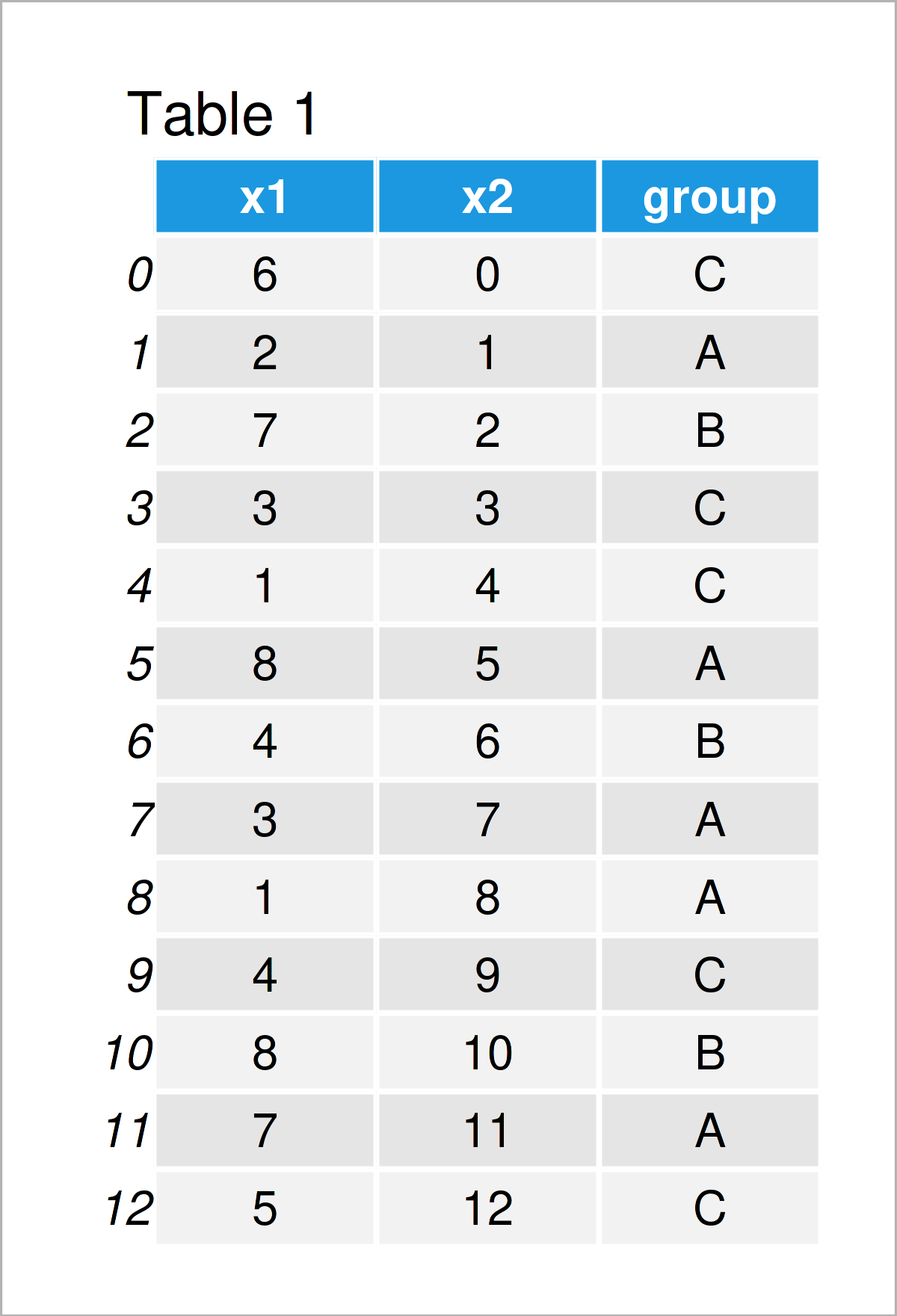# Percentile & Decile in Python (4 Examples)

On this page, I’ll show how to get the percentiles and deciles in the Python programming language.

The article consists of this information:

It’s time to dive into the exemplifying Python syntax!

## Example 1: Percentiles & Deciles of List Object

The following code illustrates how to find the percentile and decile values of a list object in Python.

As a first step, we have to create an example list:

```my_list = [8, 2, 4, 1, 3, 7, 4, 1, 3, 8, 2, 5, 3, 7] # Create example list
print(my_list)                                       # Print example list
# [8, 2, 4, 1, 3, 7, 4, 1, 3, 8, 2, 5, 3, 7]```

Furthermore, we have to import the NumPy add-on package to Python:

`import numpy as np                                   # Import NumPy library to Python`

Now, we can use the quantile and arange functions to return the percentiles of our list:

```print(np.quantile(my_list, q = np.arange(0.01, 1, 0.01)))           # Get percentiles of list
# [1.   1.   1.   1.   1.   1.   1.   1.04 1.17 1.3  1.43 1.56 1.69 1.82
#  1.95 2.   2.   2.   2.   2.   2.   2.   2.   2.12 2.25 2.38 2.51 2.64
#  2.77 2.9  3.   3.   3.   3.   3.   3.   3.   3.   3.   3.   3.   3.
#  3.   3.   3.   3.   3.11 3.24 3.37 3.5  3.63 3.76 3.89 4.   4.   4.
#  4.   4.   4.   4.   4.   4.06 4.19 4.32 4.45 4.58 4.71 4.84 4.97 5.2
#  5.46 5.72 5.98 6.24 6.5  6.76 7.   7.   7.   7.   7.   7.   7.   7.
#  7.05 7.18 7.31 7.44 7.57 7.7  7.83 7.96 8.   8.   8.   8.   8.   8.
#  8.  ]```

Similar to that, we can also compute the decile values of our list. All we have to change is the sequence specified within the arange function:

```print(np.quantile(my_list, q = np.arange(0.1, 1, 0.1)))             # Get deciles of list
# [1.3 2.  2.9 3.  3.5 4.  5.2 7.  7.7]```

## Example 2: Percentiles & Deciles of One Particular Column in pandas DataFrame

This section illustrates how to compute the percentiles and deciles of the columns in a pandas DataFrame.

Let’s first import the pandas library:

`import pandas as pd                                  # Load pandas`

Also, we have to construct an exemplifying pandas DataFrame:

```data = pd.DataFrame({'x1':[6, 2, 7, 3, 1, 8, 4, 3, 1, 4, 8, 7, 5],  # Create pandas DataFrame
'x2':range(0, 13),
'group':['C', 'A', 'B', 'C', 'C', 'A', 'B', 'A', 'A', 'C', 'B', 'A', 'C']})
print(data)                                          # Print pandas DataFrame```After executing the previous Python syntax the pandas DataFrame shown in Table 1 has been created.

In the next step, we can calculate the percentile of a particular column (i.e. x1) in this data set:

```print(data['x1'].quantile(np.arange(0.01, 1, 0.01))) # Get percentiles of one column
# 0.01    1.0
# 0.02    1.0
# 0.03    1.0
# 0.04    1.0
# 0.05    1.0
#        ...
# 0.95    8.0
# 0.96    8.0
# 0.97    8.0
# 0.98    8.0
# 0.99    8.0
# Name: x1, Length: 99, dtype: float64```

By changing the sequence within the arange function, we can also print the deciles for this column:

```print(data['x1'].quantile(np.arange(0.1, 1, 0.1)))   # Get deciles of one column
# 0.1    1.2
# 0.2    2.4
# 0.3    3.0
# 0.4    3.8
# 0.5    4.0
# 0.6    5.2
# 0.7    6.4
# 0.8    7.0
# 0.9    7.8
# Name: x1, dtype: float64```

## Example 3: Percentiles & Deciles of All Columns in pandas DataFrame

It is also possible to return the percentiles and deciles of all columns in a pandas DataFrame.

The Python syntax below calculates the percentiles for all float columns…

```print(data.quantile(np.arange(0.01, 1, 0.01)))       # Get percentiles of all columns
#        x1     x2
# 0.01  1.0   0.12
# 0.02  1.0   0.24
# 0.03  1.0   0.36
# 0.04  1.0   0.48
# 0.05  1.0   0.60
#   ...    ...
# 0.95  8.0  11.40
# 0.96  8.0  11.52
# 0.97  8.0  11.64
# 0.98  8.0  11.76
# 0.99  8.0  11.88
#
# [99 rows x 2 columns]```

…and the following code finds the deciles for the float columns in our data:

```print(data.quantile(np.arange(0.1, 1, 0.1)))         # Get deciles of all columns
#       x1    x2
# 0.1  1.2   1.2
# 0.2  2.4   2.4
# 0.3  3.0   3.6
# 0.4  3.8   4.8
# 0.5  4.0   6.0
# 0.6  5.2   7.2
# 0.7  6.4   8.4
# 0.8  7.0   9.6
# 0.9  7.8  10.8```

## Example 4: Percentiles & Deciles by Group in pandas DataFrame

Example 4 explains how to get the percentile and decile numbers by group.

To accomplish this, we have to use the groupby function in addition to the quantile function.

The following code finds the first percentile by group…

```print(data.groupby('group').quantile(0.01))          # Get first percentile by group
#          x1    x2
# group
# A      1.04  1.16
# B      4.06  2.08
# C      1.08  0.12```

…and the syntax below computes the first grouped deciles:

```print(data.groupby('group').quantile(0.1))           # Get first decile by group
#         x1   x2
# group
# A      1.4  2.6
# B      4.6  2.8
# C      1.8  1.2```

## Video & Further Resources

Would you like to know more about the calculation of the percentiles and deciles of a list and the columns of a pandas DataFrame? Then I recommend watching the following video on my YouTube channel. In the video, I’m explaining the Python codes of this article.

In addition, you might read the related tutorials on my website. I have published numerous tutorials already.

You have learned in this post how to calculate the percentiles and deciles of a list and the columns of a pandas DataFrame in the Python programming language. If you have additional questions, let me know in the comments.

Subscribe to the Statistics Globe Newsletter